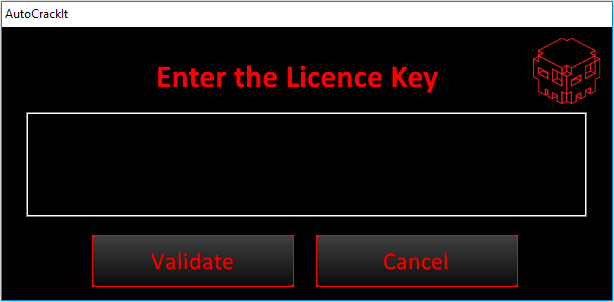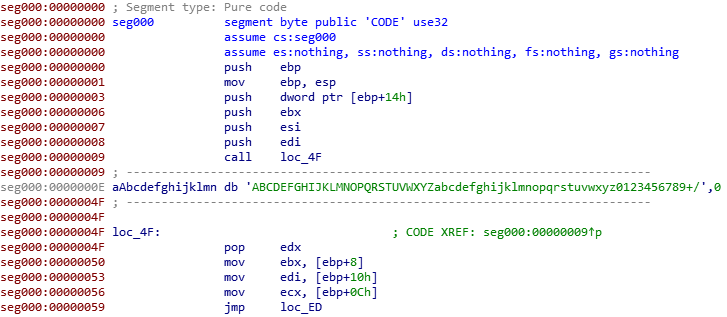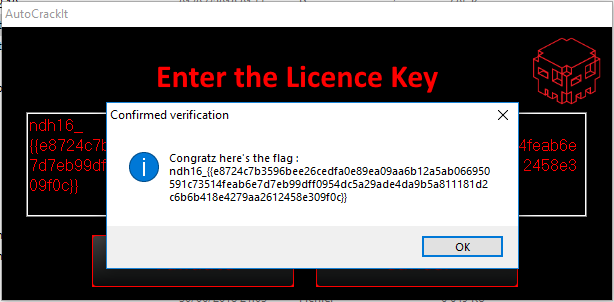# AutoCrackIt

NDH 2018 - RE (150 pts).

# NDH 2018 - AutoCrackIt

Event Challenge Category Points Solves
Nuit du hack 16 AutoCrackIt Reverse 150 ??

### Description

This is a window for a license key validator written in AutoIt. You can find the associated file here : AutoCrackIt.exe

### TL;DR

I started to decompile the executable with AutoIt3 decompiler. Then I looked for the piece of code which manages window events. The user input is manipulated by three functions and the result is compared with an encrypted license key. By executing the functions I guessed what they were doing and I wrote a script to reverse the encrypted licence key.

### Find the interesting piece of code

I began by running the executable. It’s a simple window with two buttons and an edit text.As indicated by his name, this program may be written in AutoIt. So I tried to decompile it with AutoIt3 decompiler. I looked for the string “Validate” in the decompiled source and I found the code which checks the license key.

	$licence = "9677BD05BC969C4C8602A43163FC5BA313A04A8EC59F0A14A8F3457C..." [...] While 1$nmsg = GUIGetMsg()
Switch $nmsg Case$gui_event_close
Exit
Case $cancel Exit Case$validate
If (cryyypt(cryypt(crypt(GUICtrlRead($edit1)))) ==$licence) Then
MsgBox(64, "Confirmed verification", "Congratz here's the flag : " & @CRLF & GUICtrlRead($edit1)) ClipPut(GUICtrlRead($edit1))
Else
MsgBox(16, "Failed verification", "The Licence Key is incorrect")
EndIf
EndSwitch
WEnd


### Reverse the algorithm

As you can see above the user input ($edit1) is manipulated by three functions crypt,cryypt and cryyypt. The result must be equals to$licence variable.

#### crypt

I watched the first function crypt.

Func crypt($data,$linebreak = 76)
Local $opcode = "0x5589E5FF7514535657E8410000004142434445464748494A4B4C4D4E4F50515253545556..." Local$codebuffer = DllStructCreate("byte[" & BinaryLen($opcode) & "]") DllStructSetData($codebuffer, 1, $opcode)$data = Binary($data) Local$input = DllStructCreate("byte[" & BinaryLen($data) & "]") DllStructSetData($input, 1, $data)$linebreak = Floor($linebreak / 4) * 4 Local$oputputsize = Ceiling(BinaryLen($data) * 4 / 3)$oputputsize = $oputputsize + Ceiling($oputputsize / $linebreak) * 2 + 4 Local$ouput = DllStructCreate("char[" & $oputputsize & "]") DllCall("user32.dll", "none", "CallWindowProc", "ptr", DllStructGetPtr($codebuffer), "ptr", DllStructGetPtr($input), "int", BinaryLen($data), "ptr", DllStructGetPtr($ouput), "uint",$linebreak)
Return DllStructGetData($ouput, 1) EndFunc  As you can see the function call native function CallWindowProc with a pointer to$opcode as lpPrevWndFunc. This will indirectly execute the content of $opcode variable. This is a trick often used by malware to call native code. The$data variable is stored into $input which is passed as hWnd of CallWindowProc function and consequently as hWnd of lpPrevWndFunc. So the native code ($opcode) may manipulate our input. I saved it into a file and I disassembled it with IDA.As you can see, there is a strange string “ABCDEFGHIJKLMNOPQRSTUVWXYZabcdefghijklmnopqrstuvwxyz0123456789+/” which reminded us of base64 algorithm. To confirm my hypothesis I ran the crypt function with “Hello world” as parameters.

ConsoleWrite(crypt("Hello world"))


The above code will give the following output SGVsbG8gd29ybGQ= which confirm our hypothesis that crypt function encodes the input in base64.

#### cryypt

As you can see the following function add or subtract 13 on each character of $data, it’s maybe a ROT-13 encryption algorithm. By running it I confirmed my hypothesis. Func cryypt($data)
If $data == "" Then Return$data
EndIf
Local $aarray = StringToASCIIArray($data)
For $i = 0 To UBound($aarray) - 1
If ($aarray[$i] >= 65 AND $aarray[$i] <= 77) OR ($aarray[$i] >= 97 AND $aarray[$i] <= 109) Then
$aarray[$i] += 13
ElseIf ($aarray[$i] >= 78 AND $aarray[$i] <= 90) OR ($aarray[$i] >= 110 AND $aarray[$i] <= 122) Then
$aarray[$i] -= 13
EndIf
Next
Return StringFromASCIIArray($aarray) EndFunc  #### cryyypt The cryyypt function is a little bit more complicated to guess X). Func cryyypt($data)
$i = 0$crypteddata = ""
$adata = StringSplit($data, "", 2)
For $char In$adata
If (Mod($i, 2) == 0) Then$crypteddata = $crypteddata & StringTrimLeft(_crypt_hashdata($char, 32781), 2)
Else
$crypteddata =$crypteddata & $char EndIf$i += 1
Next
Return STRINGREVERSE($crypteddata) EndFunc  _crypt_hashdata will hash the char with the selected algorithm. 32781 is the id of the SHA384 algorithm. As you can see there is a test with the counter variable$i

Mod(\$i, 2) == 0


This mean that one character out of two will be hash by SHA384 algorithm. At the end, the result string is reversed.

### Putting all the pieces together

So the license key is just a base64 encoded string which is ciphered with ROT-13 algorithm which is partially hashed. We can easily write a script to decode it (except at 4 am x) ).

First, I created a dictionary to associate characters to they corresponding reversed sha384 hash (because licence key is reversed cf cryyypt).

def sha384(s):
h = hashlib.sha384()
h.update(s)
return h.hexdigest()[::-1].upper()

correspondance = {}
for i in range(0,256):
correspondance[sha384(chr(i))] = chr(i)


Then we will attack the license key block by block.

A block is composed of one character and a sha384 hash. So the blockSize is 48 (size of a sha384 hash) * 2 (because the result is in hexadecimal, so 2 digits for 1 byte) + 1 (for the one character). For each block, we will find the corresponding characters from the reversed hash.

s=""
blockSize = 48*2+1
for i in range(0,len(licence),blockSize):
print(licence[i:i+blockSize])
c = licence[i:i+blockSize]
h = licence[i:i+blockSize][1:]
ch = correspondance[h]
s = ch + c + s


After retrieved the string I decrypted it with ROT-13 and decoded it with base64 algorithm.

print(s.decode("rot13").decode("base64"))